/

### Clustering techniques for universe selection

Example Cluster Analysis (click on chart for more details)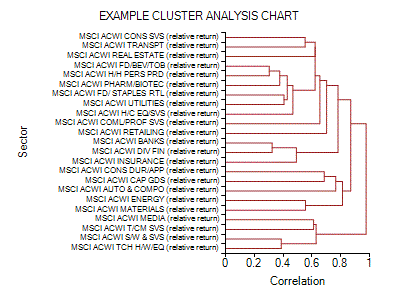1.       Cluster analysis is a well-established tool in quantitative finance. We might for example want to know which stocks appear to behave most ‘similarly’ to which other stocks, thus grouping together stocks that appear to have similar economic characteristics. Market index data vendors often classify stocks according to some predefined industry classification, but not all stocks easily fit into such classifications. Even if they did, which industry sub-types should be grouped together to form overall industry sectors? Also, is it better to analyse stocks by country first and then sector or vice-versa? Etc.

2.       Most types of cluster analysis used in finance involve hierarchical clustering. This can be thought of as a form of unsupervised learning. We have some information about individual elements and we want to build up a nested tree that best characterises the degree of linkage between the different elements (without presupposing any ‘right answer’ in advance). For example, we might have a series of stock or sector returns, and we want to see which ones appear to be closest to each other. The output is a bunch of fully nested sets. The smallest sets are the individual elements themselves. The largest set is the whole data set. The intermediate sets are nested, i.e. the intersection of any two sets is either the null set or the smaller of the two sets.

3.       The common convention is to have the nesting arrangement form a binary tree, i.e. where each larger set is deemed to split into just two sub-sets at each node of the tree. Where say three subsets are equally near each other within a larger set then this is typically represented by an arbitrary choice of one of the three subsets to stand distinct and for a branch of zero length to join it to the join of the other two subsets.

4.       For example, quantitative equity research analysts might focus on correlations between different regional sectors and correlations of stocks within sectors, computed using regression analyses over over suitable rolling periods, computing sector and country betas from the following formula, see e.g. Morgan Stanley (2002):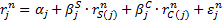where: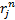is return of stockin month,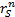is return of sector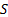in month,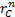is return of country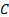in month,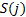and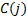are sector and country of stockand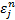is unexplained return of stockin month5.       Precise choice of how to measure ‘degree of linkage’, i.e. the ‘distance’ between different elements, can be quite important in this context, and can depend on what question we are trying to answer. For example, in an equity orientated analysis as above, we might measure ‘distance’ either by reference to correlations or by reference to covariances. If we use covariances then relatively unvolatile stocks will be deemed to be relatively similar whilst relatively volatile stocks may be deemed to be relatively different to each other even when they are relatively highly correlated. The algorithm used to derive the example cluster analysis shown above is based on one in Press et al. (2007).

References

Morgan Stanley (2002). Quantitative Strategies Research Note. Morgan Stanley

Press, W.H., Teukolsky, S.A., Vetterling, W.T. and Flannery, B.P. (2007). Numerical Recipes: The Art of Scientific Computing. Cambridge University Press